• ## 递归算法时间复杂度分析

万次阅读 多人点赞 2018-09-17 16:16:59
递归算法时间复杂度分析 时间复杂度： 一般情况下，算法中基本操作重复的次数就是问题规模n的某个函数f（n），进而分析f（n）随n的变化情况并确定T（n）的数量级。这里用‘o’来表示数量级，给出算法时间复杂度。 T...
                             递归算法时间复杂度分析
时间复杂度：
一般情况下，算法中基本操作重复的次数就是问题规模n的某个函数f（n），进而分析f（n）随n的变化情况并确定T（n）的数量级。这里用‘o’来表示数量级，给出算法时间复杂度。  T（n）=o（f（n））；  它表示随问题规模n的增大，算法的执行时间增长率和f（n）增长率成正比，这称作算法的渐进时间复杂度。而我们一般情况下讨论的最坏的时间复杂度。  空间复杂度：  算法的空间复杂度并不是实际占用的空间，而是计算整个算法空间辅助空间单元的个数，与问题的规模没有关系。算法的空间复杂度S（n）定义为该算法所耗费空间的数量级。  S（n）=o（f（n））  若算法执行所需要的辅助空间相对于输入数据n而言是一个常数，则称这个算法空间复杂度辅助空间为o（1）；  递归算法空间复杂度：递归深度n*每次递归所要的辅助空间，如果每次递归所需要的辅助空间为常数，则递归空间复杂度o（n）。
递归算法分析
1、利用数列知识
累加法：递推关系式为an+1−an=f(n)an+1−an=f(n)采用累加法。累乘法：递推关系式为an+1an=f(n)an+1an=f(n)采用累乘法。构造法：递推关系式为(1)aa+1=pan+qaa+1=pan+q，(2)aa+1=pan+qnaa+1=pan+qn，都可以通过恒等变形，构造出等差或等比数列，利用等差或等比数列的定义进行解题，其中的构造方法可通过待定系数法来进行。和化项法：递推公式为Sn=f(n)Sn=f(n)或Sn=f(an)Sn=f(an)一般利用 an={S1，Sn−Sn−1，当n=1当n>=2an={S1，当n=1Sn−Sn−1，当n>=2 用特征方程求解递推方程（感觉比较生僻，不做解释）迭代法： 从原始递推方程开始，反复将对于递推方程左边的函数用右边的等式代入，直到得到初值，然后将所得的结果进行化简。  例如在调用归并排序mergeSort(a,0,n-1)对数组a[0...n−1]a[0...n−1]排序时，执行时间T(n)T(n)的递推关系式为： T(n)={O(1)，2T(n2)+O(n)，当n=1当n>=2T(n)={O(1)，当n=12T(n2)+O(n)，当n>=2  其中，O(n)O(n)为merge()所需要的时间，设为cncn（c为正常量）。因此：  T(n)=2T(n2)+cn=2(2T(n4)+cn2)+cn=22T(n4)+2cn=23T(n8)+3cn=...=2kT(n2k)+kcn=nO(1)+cnlog2n=O(nlog2n),(假设n=2k,则k=log2n)T(n)=2T(n2)+cn=2(2T(n4)+cn2)+cn=22T(n4)+2cn=23T(n8)+3cn=...=2kT(n2k)+kcn=nO(1)+cnlog2⁡n=O(nlog2⁡n),(假设n=2k,则k=log2⁡n)

忽略求解细节。在我们求解递归式时，因为最终是要求得一个时间上限，所以在求解时常常省略一些细节。比如mergeSort(a,0,n-1)运行时间的实际递归式应该是：
T(n)={O(1)，T(⌈n2⌉)+T(⌊n2⌋)+O(n)，当n=1当n>=2T(n)={O(1)，当n=1T(⌈n2⌉)+T(⌊n2⌋)+O(n)，当n>=2
但我们忽略这些上取整、下取整以及边界条件，甚至假设问题规模n=2kn=2k，这都是为方便求解而忽略的细节。经验和一些定理告诉我们，这些细节不会影响算法时间复杂度的渐近界。

类似的，我们也可以用迭代法求解汉诺塔递归求解时的时间复杂度。但遗憾的是，迭代法一般适用于一阶的递推方程。对于二阶及以上（即T(n)依赖它前面更多个递归项T(n)依赖它前面更多个递归项）的递推方程，迭代法将导致迭代后的项太多，从而使得求和公式过于复杂，因此需要将递推方程化简，利用差消法等技巧将高阶递推方程化为一阶递推方程。如在求快速排序算法平均时间复杂度T(n)T(n)的递推方程，T(n)T(n)依赖T(n−1)、T(n−2)、...、T(1)T(n−1)、T(n−2)、...、T(1)等所有的项，这样的递推方程也称为全部历史递推方程。（这里省略快速排序算法平均复杂度T(n)的求解过程）
小结：上面6种递推关系是高中、本科知识，在此重点介绍了迭代法，其它几种方法虽未在本篇中使用，但可以加深对递推式求解的认识。

2、代入法
代入法实质上就是数学归纳法，因此求递推式分为两步：
猜测解的形式；用数学归纳法求出解中的常数，并证明解是正确的。
遗憾的是并不存在通用的方法来猜测递归式的正确解，需要凭借经验，偶尔还需要创造力。即使猜出了递归式解的渐近界，也有可能在数学归纳证明时莫名其妙的失败。正是由于该方法技术细节较为难掌握，因此这个方法不适合用来求解递归方程，反而比较适合作为其他方法检验手段。在此不做总结。可以翻阅《算法导论》进行学习。

3、递归树
递归树是一棵结点带权值的树。初始的递归树只有一个结点，它的权标记为T(n)T(n)；然后按照递归树的迭代规则不断进行迭代，每迭代一次递归树就增加一层，直到树中不再含有权值为函数的结点（即叶结点都为T(1)T(1)）。下面以递归方程
T(n)={O(1)，2T(n2)+O(n)，当n=1当n>=2;(假设n=2k,则k=log2n)T(n)={O(1)，当n=12T(n2)+O(n)，当n>=2;(假设n=2k,则k=log2⁡n)
来讲述递归树的迭代规则。

第一步： 把根结点T(n)T(n)用根是cncn、左结点为T(n2)T(n2)、右结点为T(n2)T(n2)的子树代替（即：以分解、合并子问题需要的代价为根，分解得到的子问题为叶的子树。其中常量c代表求解规模为1的问题所需的时间）；（如下如(a)→(b)(a)→(b)）第二步：把叶结点按照“第一步”的方式展开；T(n2)T(n2)用根是cn/2cn/2、左节点为T(n4)T(n4)、右结点为T(n4)T(n4)的子树代替。（如下如(b)→(c)(b)→(c)）第三步：反复按照“第一步”的方式迭代，每迭代一次递归树就增加一层，直到树中不再含有权值为函数的结点（即叶结点都为T(1)T(1)）。（如下如(c)→(d)(c)→(d)）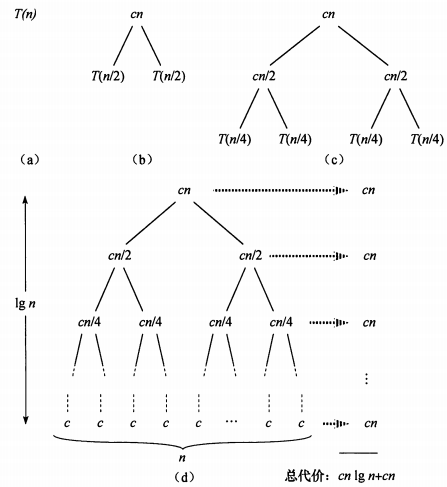在得到递归树后，将树中每层中的代价求和，得到每层代价，然后将所有层的代价求和，得到所有层次的递归调用的总代价。在上图(d)部分中，完全展开的递归树高度为lgnlg⁡n(树高为根结点到叶结点最长简单路径上边的数目)，所有递归树具有lgn+1lg⁡n+1层，所以总代价为cn∗(lgn+1)cn∗(lg⁡n+1)，所有时间复杂度为Θ(nlgn)Θ(nlg⁡n)。
总结：递归树模型求解递归方程，本质上就是迭代思想的应用，利用递归方程迭代展开过程构造对应的递归树，然后把每层的时间代价进行求和。不过递归树模型更直观，同时递归树也克服了二阶及更高阶递推方程不方便迭代展开的痛点。

4、主方法求解递推式
主方法为如下形式的递归式提供了一种“菜谱”式的求解方法，如下所示
T(n)=aT(n/b)+f(n)T(n)=aT(n/b)+f(n)

其中a≥1a≥1和b>1b>1是常数，f(n)f(n)是渐近正函数。这个递推式将规模为n的问题分解为a个子问题，每个子问题的规模为n/bn/b，a个子问题递归地求解，每个花费时间T(n/b)T(n/b)。函数f(n)f(n)包含了问题分解和子问题解合并的代价。同样，这个递归式也没有考虑上取整、下取整、边界条件等，结果不会影响递归式的渐近性质。

定理4.1(主定理) 令a≥1和b>1是常数，f(n)f(n)是一个函数，T(n)T(n)是定义在非负整数上的递归式：
T(n)=aT(n/b)+f(n)T(n)=aT(n/b)+f(n)
其中我们将n/bn/b解释为⌊n/b⌋⌊n/b⌋或⌈n/b⌉⌈n/b⌉。那么T(n)T(n)有如下渐近界：
1. 若对某个常数ε>0ε>0有f(n)=O(n(logba)−ε)f(n)=O(n(logb⁡a)−ε)，则T(n)=Θ(nlogba)T(n)=Θ(nlogb⁡a)
2. 若f(n)=Θ(nlogba)f(n)=Θ(nlogb⁡a)，则T(n)=Θ(nlogbalgn)T(n)=Θ(nlogb⁡alg⁡n)。
3. 若对某个常数ε>0ε>0有f(n)=Ω(n(logba)+ε)f(n)=Ω(n(logb⁡a)+ε)，且对某个常数c<1c<1和所有足够大的n有af(n/b)≤cf(n)af(n/b)≤cf(n)，则T(n)=Θ(f(n))T(n)=Θ(f(n))

在使用主定理之前，要比较f(n)和(nlogba)f(n)和(nlogb⁡a)的大小，这个大小不是算术意义上的大小比较，而是要在多项式意义上比较。以上三种情况在多项式意义上并未覆盖f(n)f(n)的所有可能性。情况1和情况2之间有一定间隙；情况2和情况3之间也有一定间隙。如果f(n)落在这两个间隙中，或者情况3中 正则条件不成立，就不能使用主方法来求递归式。    如在递归式：T(n)=2T(n/2)+nlgnT(n)=2T(n/2)+nlg⁡n中，因为 nlogba=n<f(n)=nlgnnlogb⁡a=n<f(n)=nlg⁡n，但是f(n)f(n)并不大于n一个多项式因子nεnε ,因为对于给定的ε>0ε>0当n足够大时，均有nε>lgnnε>lg⁡n。所以找不到这样 ε>0ε>0，该递归式落入了情况2和情况3之间的间隙，不能使用主定理。    最后给出主定理应用的几个练习题：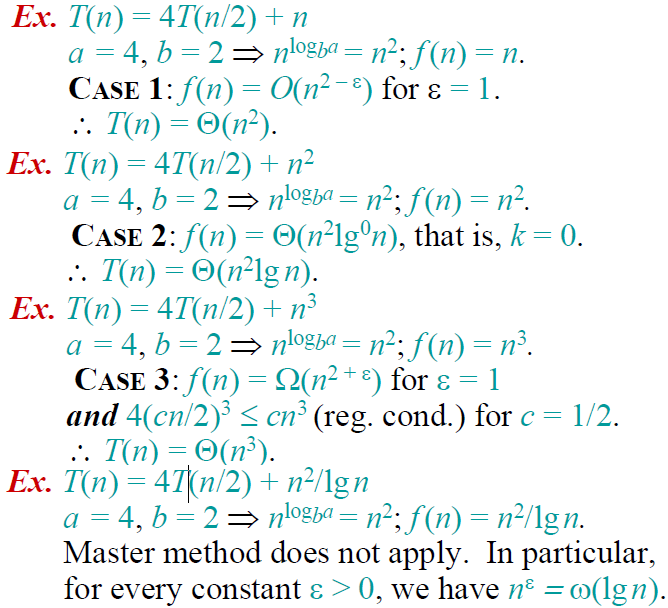具体举例分析：
【代入法】代入法首先要对这个问题的时间复杂度做出预测，然后将预测带入原来的递归方程，如果没有出现矛盾，则是可能的解，最后用数学归纳法证明。
【举   例】我们有如下的递归问题：T(n)=4T(n/2)+O(n)，我们首先预测时间复杂度为O(n2),不妨设T(n)=kn2（其中k为常数），将该结果带入方程中可得：左=kn2，右=4k(n/2)2+O(n)=kn2+O(n),由于n2的阶高于n的阶，因而左右两边是相等的，接下来用数学归纳法进行验证即可。

【迭代法】迭代法就是迭代的展开方程的右边，直到没有可以迭代的项为止，这时通过对右边的和进行估算来估计方程的解。比较适用于分治问题的求解，为方便讨论起见，给出其递归方程的一般形式：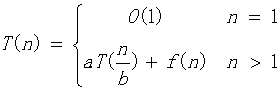【举   例】下面我们以一个简单的例子来说明：T(n)=2T(n/2)+n2,迭代过程如下：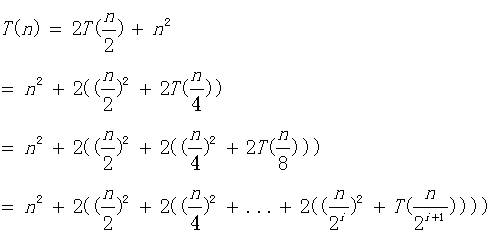容易知道，直到n/2^(i+1)=1时，递归过程结束，这时我们计算如下：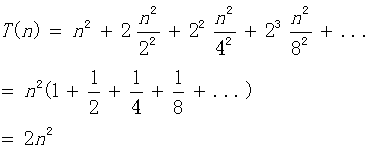到这里我们知道该算法的时间复杂度为O(n2)，上面的计算中，我们可以直接使用无穷等比数列的公式，不用考虑项数i的约束，实际上这两种方法计算的结果是完全等价的，有兴趣的同学可以自行验证。

【公式法】这个方法针对形如：T(n) = aT(n/b) + f(n)的递归方程。这种递归方程是分治法的时间复杂性所满足的递归关系，即一个规模为n的问题被分成规模均为n/b的a个子问题，递归地求解这a个子问题，然后通过对这a个子问题的解的综合，得到原问题的解。这种方法是对于分治问题最好的解法，我们先给出如下的公式：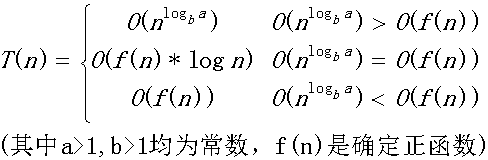公式记忆：我们实际上是比较n^logba和f(n)的阶，如果他们不等，那么T(n)取他们中的较大者，如果他们的阶相等，那么我们就将他们的任意一个乘以logn就可以了。按照这个公式，我们可以计算【迭代法】中提到的例子：O(f(n))=O(n2),容易计算另外一个的阶是O(n),他们不等，所以取较大的阶O(n2)。太简单了，不是吗？
需要注意：上面的公式并不包含所有的情况，比如第一种和第二种情况之间并不包含下面这种情况：f(n)是小于前者，但是并不是多项式的小于前者。同样后两种的情况也并不包含所有的情况。为了好理解与运用的情况下，笔者将公式表述成如上的情况，但是并不是很严谨，关于该公式的严密讨论，请看这里。但是公式的不包含的情况，我们很少很少碰到，上面的公式适用范围很广泛的。
特别地，对于我们经常碰到的，当f(n)=0时，我们有：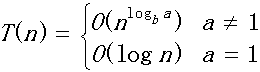【母函数法】母函数是用于对应于一个无穷序列的幂级数。这里我们解决的递归问题是形如：T(n)=c1T(n-1)+c2T(n-2)+c3T(n-3)+...+ckT(n-k)+f(n)。为说明问题简便起见，我们选择斐波那契数列的时间复杂度作为例子进行讨论。
【举  例】斐波那契数列递归公式：T(n)=T(n-1)+T(n-2)。这里我们假设F(n)为第n项的运算量。则容易得到：F(n)=F(n-1)+F(n-2),其中F(1)=F(2)=1.我们构造如下的母函数：G(x)=F(1)x+F(2)x2+F(3)x3+......,我们可以推导如下：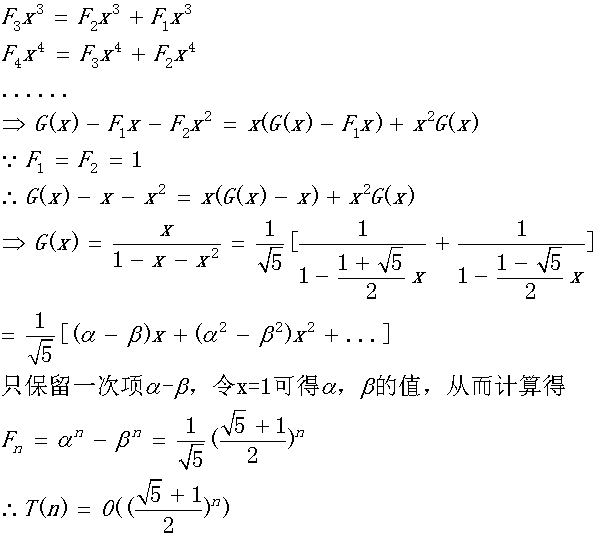上面的方法计算相对来说是比较简单的，关键在于对于母函数的理解，刚开始的时候可能不是很好理解，对于母函数可以参考这里和维基百科这里。

【差分方程法】可以将某些递归方程看成差分方程，通过解差分方程的方法来解递归方程，然后对解作出渐近阶估计。这里我们只考虑最长常见的递归形式，形如：T(n)=c1T(n-1)+c2T(n-2)+c3T(n-3)+...+ckT(n-k)+f(n)，其中c1,c2,...ck为常数且不等于0；我们对该方程的求解如下：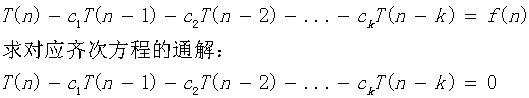对应上面的齐次方程的特征方程为：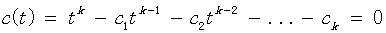如果解得t=r是该特征方程的m重根，则这m个解的形式为：{rn     n*rn      n2rn   ...    nm-1rn},其余的关于复数解的形式和普通的线性方程组的形式类似，不再赘述。接下来，我们要求解该方程的对应非齐次方程组的通解，这里我们针对该方程的特殊形式，不加证明地给出如下的通解形式：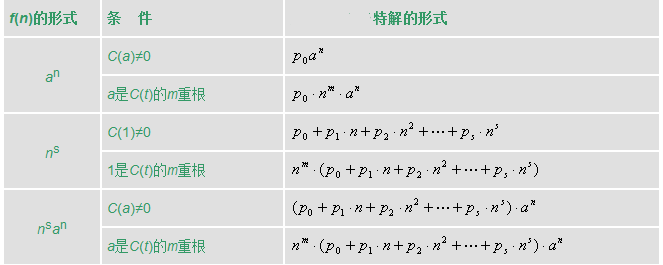则和线性代数中的解一样，原方程的解等于齐次方程组的通解+特解，即：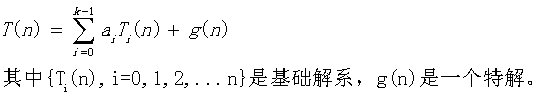最后由初始条件确定a(i)的值即可。
为了帮助理解，我们举两个例子看看，就明白是怎么回事了。
【举 例1】递归方程如下：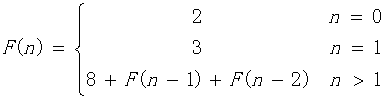(1)写出对应齐次方程的特征方程：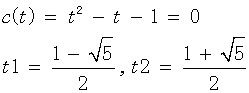得到基础解系为：{t1n,  t2n}
(2)计算特解，对于本题，直接观察得特解为：-8
(3)得到原方程解的形式为：T(n)=a0t1n+a1t2n-8
(4)代入n=0，n=1的情况，得到a0,a1，最后可得: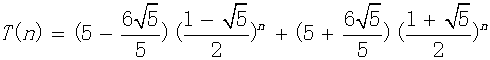可以看到该方程形式和上面讨论过的斐波那契数列只差一个常数8，因而两者的时间复杂度是相同的。有兴趣的同学可以按照这个方法再次计算斐波那契数列的时间复杂度验证一下。
【举  例2】递归方程如下：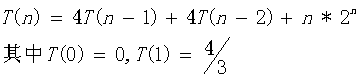（1）计算对应齐次方程的基础解析：特征方程为：C(t)=t^2-4t-4=0,得到一个2重根t=2.因而其基础解为：{2n      n*2n}
（2）由于f(n)=n*2n，对应上面表格的最后一种情况，得到特解形式为：T(n)=n2(p0+p1n)2n代入原递归方程可得：p0=1/2,p1=1/6
（3）原方程解的形式为：T(n)=a0*2n+a1*n*2n+n2(1/2+n/6)2n,代入T(0),T(1)得：a0=a1=0
（4）综上：T(n)=n2(1/2+n/6)2n
因而时间复杂度为：O(n32n).

参考：https://blog.csdn.net/so_geili/article/details/53444816
https://blog.csdn.net/m0_37734999/article/details/78751882
https://www.cnblogs.com/hlongch/p/5749038.html
展开全文• 算法 时间复杂度 空间复杂度 经典算法 时间复杂度 空间复杂度 经典算法 时间复杂度 空间复杂度 经典
• 算法复杂度分为时间复杂度和空间复杂度。 时间复杂度用于度量算法执行的时间长短；而空间复杂度则是用于度量算法所需存储空间的大小。 目录 时间复杂度 1.时间频度 2.计算方法 3.分类 空间复杂度 算法时间...
文章地址：http://lzw.me/a/algorithm-complexity.html
算法复杂度分为时间复杂度和空间复杂度。 时间复杂度用于度量算法执行的时间长短；而空间复杂度则是用于度量算法所需存储空间的大小。
目录
时间复杂度
1.时间频度
2.计算方法
3.分类
空间复杂度
算法的时间复杂度（计算实例）
算法复杂度的渐近表示法
一  大O记号
二  Ω记号
三  Θ记号
四  小o记号
五  例子
常见排序算法时空复杂度

时间复杂度
1.时间频度
一个算法执行所耗费的时间，从理论上是不能算出来的，必须上机运行测试才能知道。但我们不可能也没有必要对每个算法都上机测试，只需知道哪个算法花费的时间多，哪个算法花费的时间少就可以了。并且一个算法花费的时间与算法中语句的执行次数成正比例，哪个算法中语句执行次数多，它花费时间就多。一个算法中的语句执行次数称为语句频度或时间频度。记为T(n)。
2.计算方法
1. 一般情况下，算法的基本操作重复执行的次数是模块n的某一个函数f（n），因此，算法的时间复杂度记做：T（n）=O（f（n）） 　　分析：随着模块n的增大，算法执行的时间的增长率和f（n）的增长率成正比，所以f（n）越小，算法的时间复杂度越低，算法的效率越高。
2. 在计算时间复杂度的时候，先找出算法的基本操作，然后根据相应的各语句确定它的执行次数，再找出T（n）的同数量级（它的同数量级有以下：1，Log2n ，n ，nLog2n ，n的平方，n的三次方，2的n次方，n！），找出后，f（n）=该数量级，若T(n)/f(n)求极限可得到一常数c，则时间复杂度T（n）=O（f（n））
例：算法：

for（i=1;i<=n;++i） 　　{ 　　for(j=1;j<=n;++j) 　　{ 　　c[ i ][ j ]=0; //该步骤属于基本操作执行次数：n的平方 次 　　for(k=1;k<=n;++k) 　　c[ i ][ j ]+=a[ i ][ k ]*b[ k ][ j ]; //该步骤属于基本操作 执行次数：n的三次方 次 　　} 　　}

则有 T（n）= n的平方+n的三次方，根据上面括号里的同数量级，我们可以确定 n的三次方 为T（n）的同数量级 　　则有f（n）= n的三次方，然后根据T（n）/f（n）求极限可得到常数c 　　则该算法的 时间复杂度：T（n）=O（n的三次方）
3.分类
按数量级递增排列，常见的时间复杂度有： 　　常数阶O(1),对数阶O(log2n),线性阶O(n), 　　线性对数阶O(nlog2n),平方阶O(n2)，立方阶O(n3),…， 　　k次方阶O(nk), 指数阶O(2n) 。随着问题规模n的不断增大，上述时间复杂度不断增大，算法的执行效率越低。
空间复杂度
与时间复杂度类似，空间复杂度是指算法在计算机内执行时所需存储空间的度量。记作: 　　S(n)=O(f(n)) 　　我们一般所讨论的是除正常占用内存开销外的辅助存储单元规模。
算法的时间复杂度（计算实例）
算法的时间复杂度 定义：如果一个问题的规模是n，解这一问题的某一算法所需要的时间为T(n)，它是n的某一函数 T(n)称为这一算法的“时间复杂性”。
当输入量n逐渐加大时，时间复杂性的极限情形称为算法的“渐近时间复杂性”。
我们常用大O表示法表示时间复杂性，注意它是某一个算法的时间复杂性。大O表示只是说有上界，由定义如果f(n)=O(n)，那显然成立f(n)=O(n^2)，它给你一个上界，但并不是上确界，但人们在表示的时候一般都习惯表示前者。
此外，一个问题本身也有它的复杂性，如果某个算法的复杂性到达了这个问题复杂性的下界，那就称这样的算法是最佳算法。
“大O记法”：在这种描述中使用的基本参数是 n，即问题实例的规模，把复杂性或运行时间表达为n的函数。这里的“O”表示量级 (order)，比如说“二分检索是 O(logn)的”,也就是说它需要“通过logn量级的步骤去检索一个规模为n的数组”记法 O ( f(n) )表示当 n增大时，运行时间至多将以正比于 f(n)的速度增长。
这种渐进估计对算法的理论分析和大致比较是非常有价值的，但在实践中细节也可能造成差异。例如，一个低附加代价的O(n2)算法在n较小的情况下可能比一个高附加代价的 O(nlogn)算法运行得更快。当然，随着n足够大以后，具有较慢上升函数的算法必然工作得更快。
O(1)
Temp=i;i=j;j=temp;
以上三条单个语句的频度均为1，该程序段的执行时间是一个与问题规模n无关的常数。算法的时间复杂度为常数阶，记作T(n)=O(1)。如果算法的执行时间不随着问题规模n的增加而增长，即使算法中有上千条语句，其执行时间也不过是一个较大的常数。此类算法的时间复杂度是O(1)。
O(n^2)
2.1. 交换i和j的内容

sum=0； （一次） for(i=1;i<=n;i++) （n次） for(j=1;j<=n;j++) （n^2次） sum++； （n^2次）

解：T(n)=2n^2+n+1 =O(n^2)
2.2.

for (i=1;i<n;i++) { y=y+1; ①  for (j=0;j<=(2*n);j++)  x++; ②  }

解：语句1的频度是n-1 语句2的频度是(n-1)*(2n+1)=2n^2-n-1 f(n)=2n^2-n-1+(n-1)=2n^2-2 该程序的时间复杂度T(n)=O(n^2).
O(n)

2.3. a=0; b=1; ① for (i=1;i<=n;i++) ② {  s=a+b;　　　　③ b=a;　　　　　④  a=s;　　　　　⑤ }

解：语句1的频度：2,  语句2的频度： n,  语句3的频度： n-1,  语句4的频度：n-1,  语句5的频度：n-1,  T(n)=2+n+3(n-1)=4n-1=O(n).
O(log2n )
2.4.

i=1; ① while (i<=n) i=i*2; ②

解： 语句1的频度是1,  设语句2的频度是f(n), 则：2^f(n)<=n;f(n)<=log2n  取最大值f(n)= log2n, T(n)=O(log2n )
O(n^3)
2.5.

for(i=0;i<n;i++) {  for(j=0;j<i;j++)  { for(k=0;k<j;k++) x=x+2;  } }

解：当i=m, j=k的时候,内层循环的次数为k当i=m时, j 可以取 0,1,…,m-1 , 所以这里最内循环共进行了0+1+…+m-1=(m-1)m/2次所以,i从0取到n, 则循环共进行了: 0+(1-1)*1/2+…+(n-1)n/2=n(n+1)(n-1)/6所以时间复杂度为O(n^3).
我们还应该区分算法的最坏情况的行为和期望行为。如快速排序的最坏情况运行时间是 O(n^2)，但期望时间是 O(nlogn)。通过每次都仔细地选择基准值，我们有可能把平方情况 (即O(n^2)情况)的概率减小到几乎等于 0。在实际中，精心实现的快速排序一般都能以 (O(nlogn)时间运行。
下面是一些常用的记法：
访问数组中的元素是常数时间操作，或说O(1)操作。一个算法如 果能在每个步骤去掉一半数据元素，如二分检索，通常它就取 O(logn)时间。用strcmp比较两个具有n个字符的串需要O(n)时间。常规的矩阵乘算法是O(n^3)，因为算出每个元素都需要将n对 元素相乘并加到一起，所有元素的个数是n^2。
指数时间算法通常来源于需要求出所有可能结果。例如，n个元 素的集合共有2n个子集,所以要求出所有子集的算法将是O(2n)的。指数算法一般说来是太复杂了，除非n的值非常小，因为，在 这个问题中增加一个元素就导致运行时间加倍。不幸的是，确实有许多问题 (如著名的“巡回售货员问题” )，到目前为止找到的算法都是指数的。如果我们真的遇到这种情况，通常应该用寻找近似最佳结果的算法替代之。
算法复杂度的渐近表示法
一个算法的时间复杂度，指算法运行的时间。
假设数据输入规模是n，算法的复杂度可以表示为f(n)的函数
一  大O记号
假设f(n)和g(n)的定义域是非负整数，存在两个正整数c和n0，使得n>n0的时候，f(n)≤c*g(n),则f(n)=O(g(n))。可见O(g(n))可以表示算法运行时间的上界。O(g(n))表示的函数集合的函数是阶数不超过g(n)的函数。
例如：f(n)=2*n+2=O(n)
证明：当n>3的时候，2*n +2<3n，所以可选n0=3,c=3，则n>n0的时候，f(n)<c*(n)，所以f(n)=O(n)。
现在再证明f(n)=2*n+2=O(n^2)
证明：当n>2的时候，2*n+2<2*n^2，所以可选n0=2,c=2,则n>n0的时候，f(n)<c*(n^2)，所以f(n)=O(n^2)。
同理可证f(n)=O(n^a)，a>1
二  Ω记号
Ω记号与大O记号相反，他可以表示算法运行时间的下界。Ω（g（n））表示的函数集合的函数是所有阶数超过g(n)的函数。
例如：f(n)=2*n^2+3*n+2=Ω(n^2)
证明：当n>4的时候，2*n^2+3*n+2>n^2，所以可选n0=4,c=1,则n>n0的时候，f(n)>c*(n^2)，所以f(n)=Ω(n^2)。
同理可证f(n)=Ω(n),f(n)=Ω(1)
三  Θ记号
Θ记号介于大O记号和Ω记号之间。他表示，存在正常数c1,c2,n0，当n>n0的时候，c1*g(n)≤f(n)≤c2*g(n)，则f(n)=Θ(g(n))。他表示所有阶数与g(n)相同的函数集合。
四  小o记号
f(n)=o(g(n))当且仅当f(n)=O(g(n))且f(n)≠Ω(g(n))。也就是说小o记号可以表示时间复杂度的上界，但是一定不等于下界。
五  例子
假设f(n)=2n^2+3n+5，
则f(n)=O(n^2)或者f(n) = O(n^3)或者f(n)=O(n^4)或者……
f(n)=Ω(n^2)或者f(n)=Ω(n)或者f(n)=Ω(1)
f(n)=Θ(n^2)
f(n) = o(n^3)或者f(n)=o(n^4)或者f(n)=o(n^5)或者……
注：n^2表示n的平方，以此类推。
常见排序算法时空复杂度

排序法 最差时间分析平均时间复杂度稳定度空间复杂度冒泡排序O(n2)O(n2)稳定O(1)快速排序O(n2)O(n*log2n)不稳定O(log2n)~O(n)选择排序O(n2)O(n2)稳定O(1)二叉树排序O(n2)O(n*log2n)不一顶O(n) 插入排序 O(n2)O(n2)稳定O(1)堆排序O(n*log2n)O(n*log2n)不稳定O(1)希尔排序OO不稳定O(1)
展开全文计算机领域 复杂度 空间复杂度
• 根号n段归并排序算法时间复杂度分析过程： 1.合并 根号n向下取整 段子数组使用的是自底向上两两归并的策略 2.根号n段归并排序算法时间复杂度的数学推导
•代码
• 数据结构与算法导论1--算法时间复杂度和空间复杂度.pdf
• 平均时间复杂度 最坏时间复杂度 空间复杂度 是否稳定 冒泡排序 :————-: :—–: :—–: :—–: 选择排序 :————-: :—–: :—–: :—–: 直接插入排序 :————-: :—–: :—–: :—–: ...
排序算法分类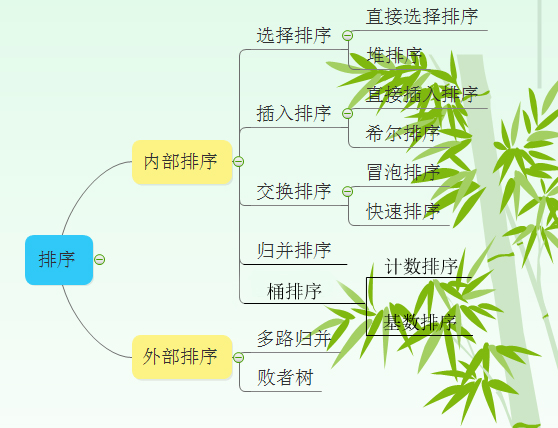排序算法比较表格填空
排序算法平均时间复杂度最坏时间复杂度空间复杂度是否稳定冒泡排序:————-::—–::—–::—–:选择排序:————-::—–::—–::—–:直接插入排序:————-::—–::—–::—–:归并排序:————-::—–::—–::—–:快速排序:————-::—–::—–::—–:堆排序:————-::—–::—–::—–:希尔排序:————-::—–::—–::—–:计数排序:————-::—–::—–::—–:基数排序:————-::—–::—–::—–:
排序算法比较表格
排序算法平均时间复杂度最坏时间复杂度空间复杂度是否稳定冒泡排序

O（n2）
$O（n^2）$

O（n2）
$O（n^2）$

O（1）
$O（1）$是选择排序

O（n2）
$O（n^2）$

O（n2）
$O（n^2）$

O（1）
$O（1）$不是直接插入排序

O（n2）
$O（n^2）$

O（n2）
$O（n^2）$

O（1）
$O（1）$是归并排序

O(nlogn)
$O(nlogn)$

O(nlogn)
$O(nlogn)$

O（n）
$O（n）$是快速排序

O(nlogn)
$O(nlogn)$

O（n2）
$O（n^2）$

O（logn）
$O（logn）$不是堆排序

O(nlogn)
$O(nlogn)$

O(nlogn)
$O(nlogn)$

O（1）
$O（1）$不是希尔排序

O(nlogn)
$O(nlogn)$

O（ns）
$O（n^s）$

O（1）
$O（1）$不是计数排序

O(n+k)
$O(n+k)$

O(n+k)
$O(n+k)$

O(n+k)
$O(n+k)$是基数排序

O(N∗M)
$O(N*M)$

O(N∗M)
$O(N*M)$

O(M)
$O(M)$是
注：
1 归并排序可以通过手摇算法将空间复杂度降到O（1），但是时间复杂度会提高。
2 基数排序时间复杂度为O（N*M），其中N为数据个数，M为数据位数。
辅助记忆
时间复杂度记忆-
冒泡、选择、直接 排序需要两个for循环，每次只关注一个元素，平均时间复杂度为

O（n2）
$O（n^2）$（一遍找元素

O(n)
$O(n)$，一遍找位置

O(n)
$O(n)$）快速、归并、希尔、堆基于二分思想，log以2为底，平均时间复杂度为

O(nlogn)
$O(nlogn)$（一遍找元素

O(n)
$O(n)$，一遍找位置

O(logn)
$O(logn)$）稳定性记忆-“快希选堆”（快牺牲稳定性）
排序算法的稳定性：排序前后相同元素的相对位置不变，则称排序算法是稳定的；否则排序算法是不稳定的。
原理理解
1 冒泡排序
1.1 过程
冒泡排序从小到大排序：一开始交换的区间为0~N-1，将第1个数和第2个数进行比较，前面大于后面，交换两个数，否则不交换。再比较第2个数和第三个数，前面大于后面，交换两个数否则不交换。依次进行，最大的数会放在数组最后的位置。然后将范围变为0~N-2，数组第二大的数会放在数组倒数第二的位置。依次进行整个交换过程，最后范围只剩一个数时数组即为有序。
1.2 动图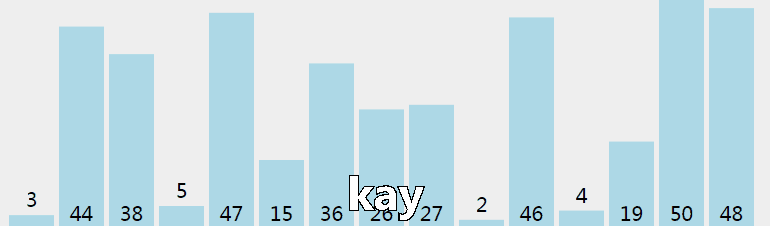1.3 核心代码（函数）
//array[]为待排序数组，n为数组长度
void BubbleSort(int array[], int n)
{
int i, j, k;
for(i=0; i<n-1; i++)
for(j=0; j<n-1-i; j++)
{
if(array[j]>array[j+1])
{
k=array[j];
array[j]=array[j+1];
array[j+1]=k;
}
}
}
2 选择排序
2.1 过程
选择排序从小到大排序：一开始从0~n-1区间上选择一个最小值，将其放在位置0上，然后在1~n-1范围上选取最小值放在位置1上。重复过程直到剩下最后一个元素，数组即为有序。
2.2 动图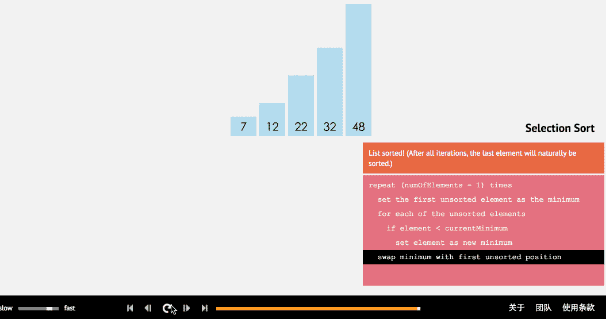2.3 核心代码（函数）
//array[]为待排序数组，n为数组长度
void selectSort(int array[], int n)
{
int i, j ,min ,k;
for( i=0; i<n-1; i++)
{
min=i; //每趟排序最小值先等于第一个数，遍历剩下的数
for( j=i+1; j<n; j++) //从i下一个数开始检查
{
if(array[min]>array[j])
{
min=j;
}
}
if(min!=i)
{
k=array[min];
array[min]=array[i];
array[i]=k;
}
}
}
3 插入排序
3.1 过程
插入排序从小到大排序：首先位置1上的数和位置0上的数进行比较，如果位置1上的数大于位置0上的数，将位置0上的数向后移一位，将1插入到0位置，否则不处理。位置k上的数和之前的数依次进行比较，如果位置K上的数更大，将之前的数向后移位，最后将位置k上的数插入不满足条件点，反之不处理。
3.2 动图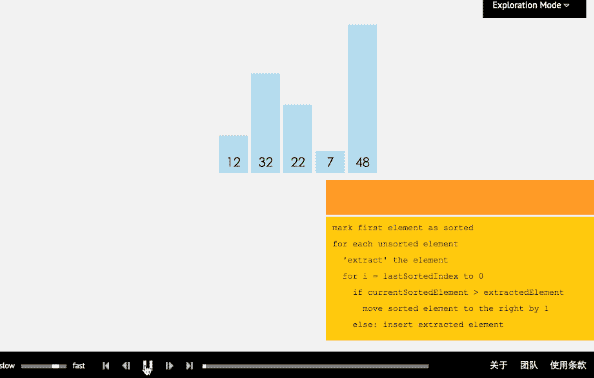3.3 核心代码（函数）
//array[]为待排序数组，n为数组长度
void insertSort(int array[], int n)
{
int i,j,temp;
for( i=1;i<n;i++)
{
if(array[i]<array[i-1])
{
temp=array[i];
for( j=i;array[j-1]>temp;j--)
{
array[j]=array[j-1];
}
array[j]=temp;
}
}
}
4 归并排序
4.1 过程
归并排序从小到大排序：首先让数组中的每一个数单独成为长度为1的区间，然后两两一组有序合并，得到长度为2的有序区间，依次进行，直到合成整个区间。
4.2 动图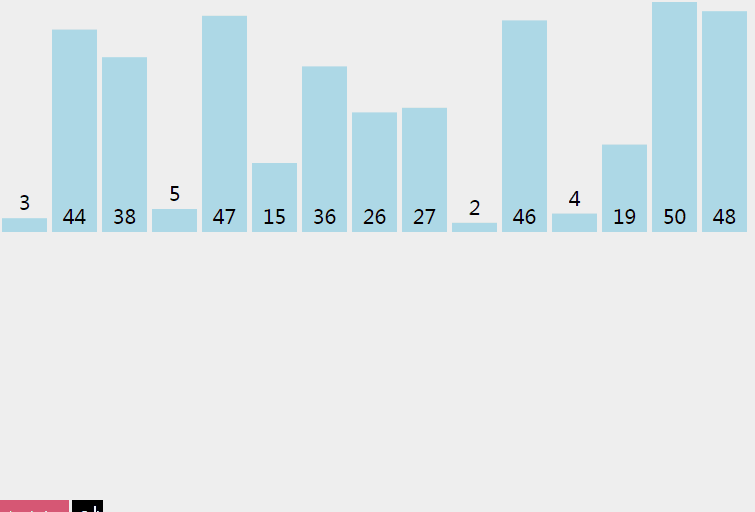4.3 核心代码（函数）
递归实现
实现归并，并把数据都放在list1里面
void merging(int *list1, int list1_size, int *list2,  int list2_size)
{
int i=0, j=0, k=0, m=0;
int temp[MAXSIZE];

while(i < list1_size && j < list2_size)
{
if(list1[i]<list2[j])
{
temp[k++] = list1[i++];
}
else
{
temp[k++] = list2[j++];
}
}
while(i<list1_size)
{
temp[k++] = list1[i++];
}
while(j<list2_size)
{
temp[k++] = list2[j++];
}

for(m=0; m < (list1_size+list2_size); m++)
{
list1[m]=temp[m];
}
}
//如果有剩下的，那么说明就是它是比前面的数组都大的，直接加入就可以了
void mergeSort(int array[], int n)
{
if(n>1)
{
int *list1 = array;
int list1_size = n/2;
int *list2 = array + n/2;
int list2_size = n-list1_size;

mergeSort(list1, list1_size);
mergeSort(list2, list2_size);

merging(list1, list1_size, list2, list2_size);
}
}
//归并排序复杂度分析：一趟归并需要将待排序列中的所有记录
//扫描一遍，因此耗费时间为O(n),而由完全二叉树的深度可知，
//整个归并排序需要进行[log2n],因此，总的时间复杂度为
//O(nlogn),而且这是归并排序算法中平均的时间性能
//空间复杂度：由于归并过程中需要与原始记录序列同样数量级的
//存储空间去存放归并结果及递归深度为log2N的栈空间，因此空间
//复杂度为O(n+logN)
//也就是说，归并排序是一种比较占内存，但却效率高且稳定的算法 
迭代实现
void MergeSort(int k[],int n)
{
int i,next,left_min,left_max,right_min,right_max;
//动态申请一个与原来数组一样大小的空间用来存储
int *temp = (int *)malloc(n * sizeof(int));
//逐级上升，第一次比较2个，第二次比较4个，第三次比较8个。。。
for(i=1; i<n; i*=2)
{
//每次都从0开始，数组的头元素开始
for(left_min=0; left_min<n-i; left_min = right_max)
{
right_min = left_max = left_min + i;
right_max = left_max + i;
//右边的下标最大值只能为n
if(right_max>n)
{
right_max = n;
}
//next是用来标志temp数组下标的，由于每次数据都有返回到K，
//故每次开始得重新置零
next = 0;
//如果左边的数据还没达到分割线且右边的数组没到达分割线，开始循环
while(left_min<left_max&&right_min<right_max)
{
if(k[left_min] < k[right_min])
{
temp[next++] = k[left_min++];
}
else
{
temp[next++] = k[right_min++];
}
}
//上面循环结束的条件有两个，如果是左边的游标尚未到达，那么需要把
//数组接回去，可能会有疑问，那如果右边的没到达呢，其实模拟一下就可以
//知道，如果右边没到达，那么说明右边的数据比较大，这时也就不用移动位置了

while(left_min < left_max)
{
//如果left_min小于left_max，说明现在左边的数据比较大
//直接把它们接到数组的min之前就行
k[--right_min] = k[--left_max];
}
while(next>0)
{
//把排好序的那部分数组返回该k
k[--right_min] = temp[--next];
}
}
}
}
//非递归的方法，避免了递归时深度为log2N的栈空间，
//空间只是用到归并临时申请的跟原来数组一样大小的空间，并且在时间性能上也有一定的提升，
//因此，使用归并排序是，尽量考虑用非递归的方法。
5 快速排序
5.1 过程
快速排序从小到大排序：在数组中随机选一个数（默认数组首个元素），数组中小于等于此数的放在左边，大于此数的放在右边，再对数组两边递归调用快速排序，重复这个过程。
5.2 动图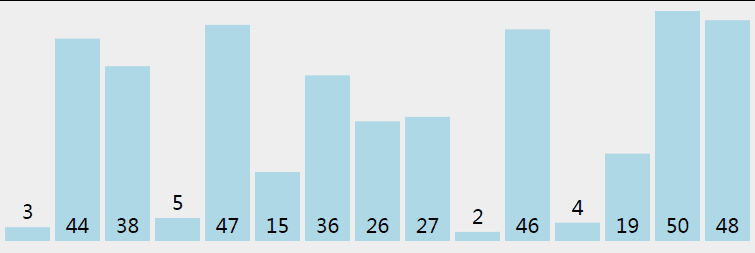5.3 核心代码（函数）
推荐程序（好理解）
//接口调整
{
quicksort(k,0,n-1);
}
void quicksort(int a[], int left, int right)
{
int i,j,t,temp;
if(left>right)   //（递归过程先写结束条件）
return;

temp=a[left]; //temp中存的就是基准数
i=left;
j=right;
while(i!=j)
{
//顺序很重要，要先从右边开始找（最后交换基准时换过去的数要保证比基准小，因为基准
//选取数组第一个数，在小数堆中）
while(a[j]>=temp && i<j)
j--;
//再找右边的
while(a[i]<=temp && i<j)
i++;
//交换两个数在数组中的位置
if(i<j)
{
t=a[i];
a[i]=a[j];
a[j]=t;
}
}
//最终将基准数归位 （之前已经temp=a[left]过了，交换只需要再进行两步）
a[left]=a[i];
a[i]=temp;

quicksort(left,i-1);//继续处理左边的，这里是一个递归的过程
quicksort(i+1,right);//继续处理右边的 ，这里是一个递归的过程
}  
6 堆排序
6.1 过程
堆排序从小到大排序：首先将数组元素建成大小为n的大顶堆，堆顶（数组第一个元素）是所有元素中的最大值，将堆顶元素和数组最后一个元素进行交换，再将除了最后一个数的n-1个元素建立成大顶堆，再将最大元素和数组倒数第二个元素进行交换，重复直至堆大小减为1。
注：完全二叉树  假设二叉树深度为n，除了第n层外，n-1层节点都有两个孩子，第n层节点连续从左到右。如下图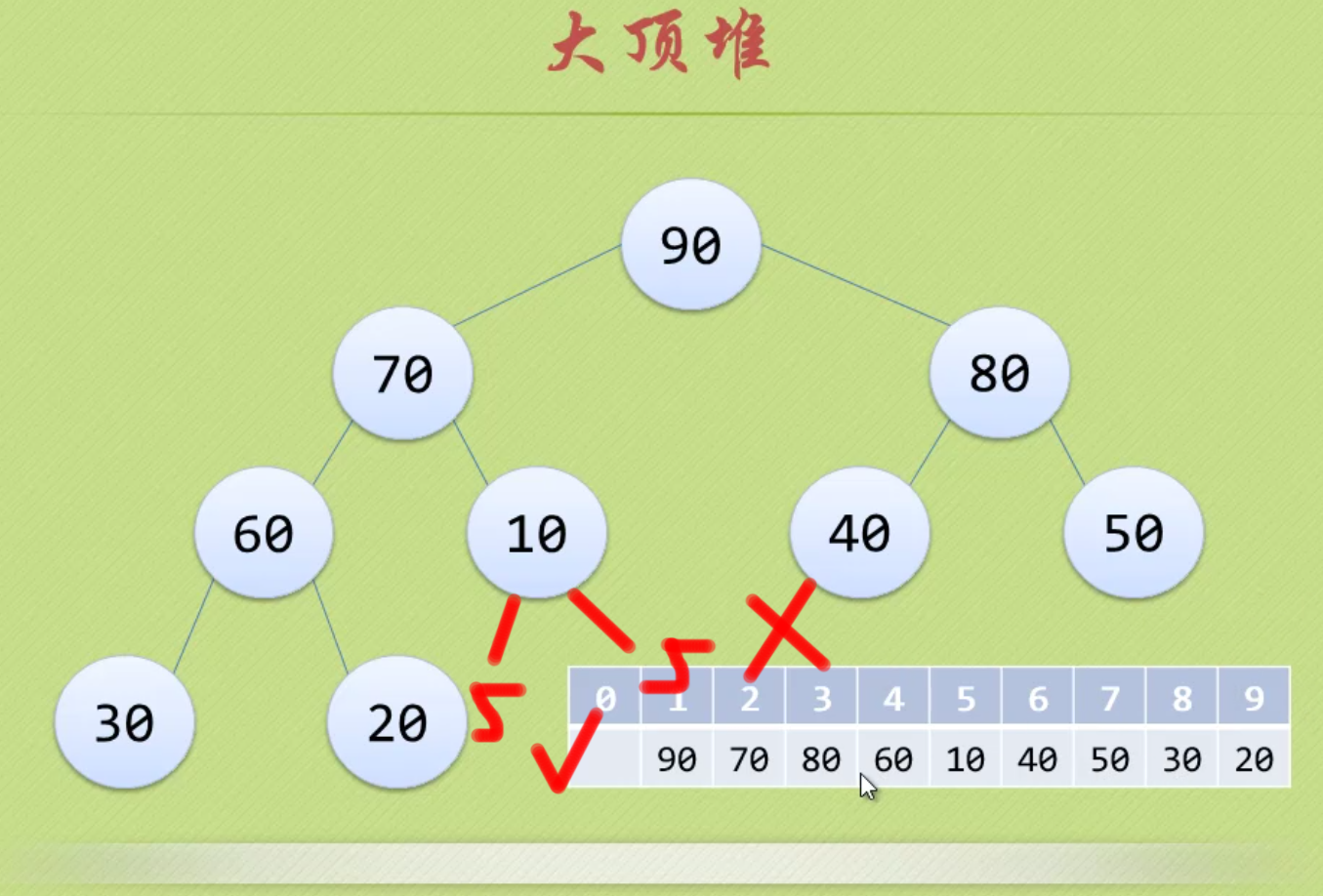注：大顶堆  大顶堆是具有以下性质的完全二叉树：每个节点的值都大于或等于其左右孩子节点的值。  即，根节点是堆中最大的值，按照层序遍历给节点从1开始编号，则节点之间满足如下关系：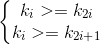(1<=i<=n/2)
6.2 动图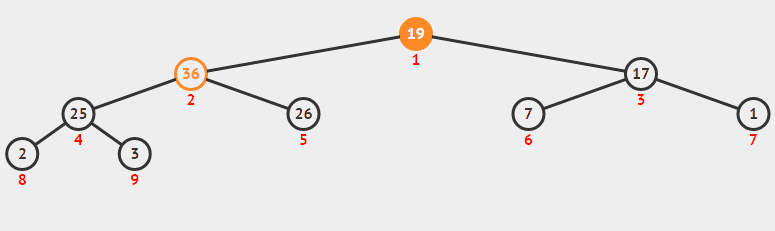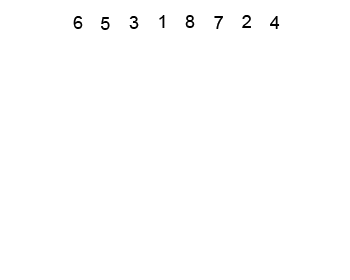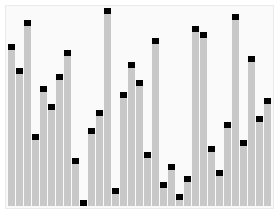6.3 核心代码（函数）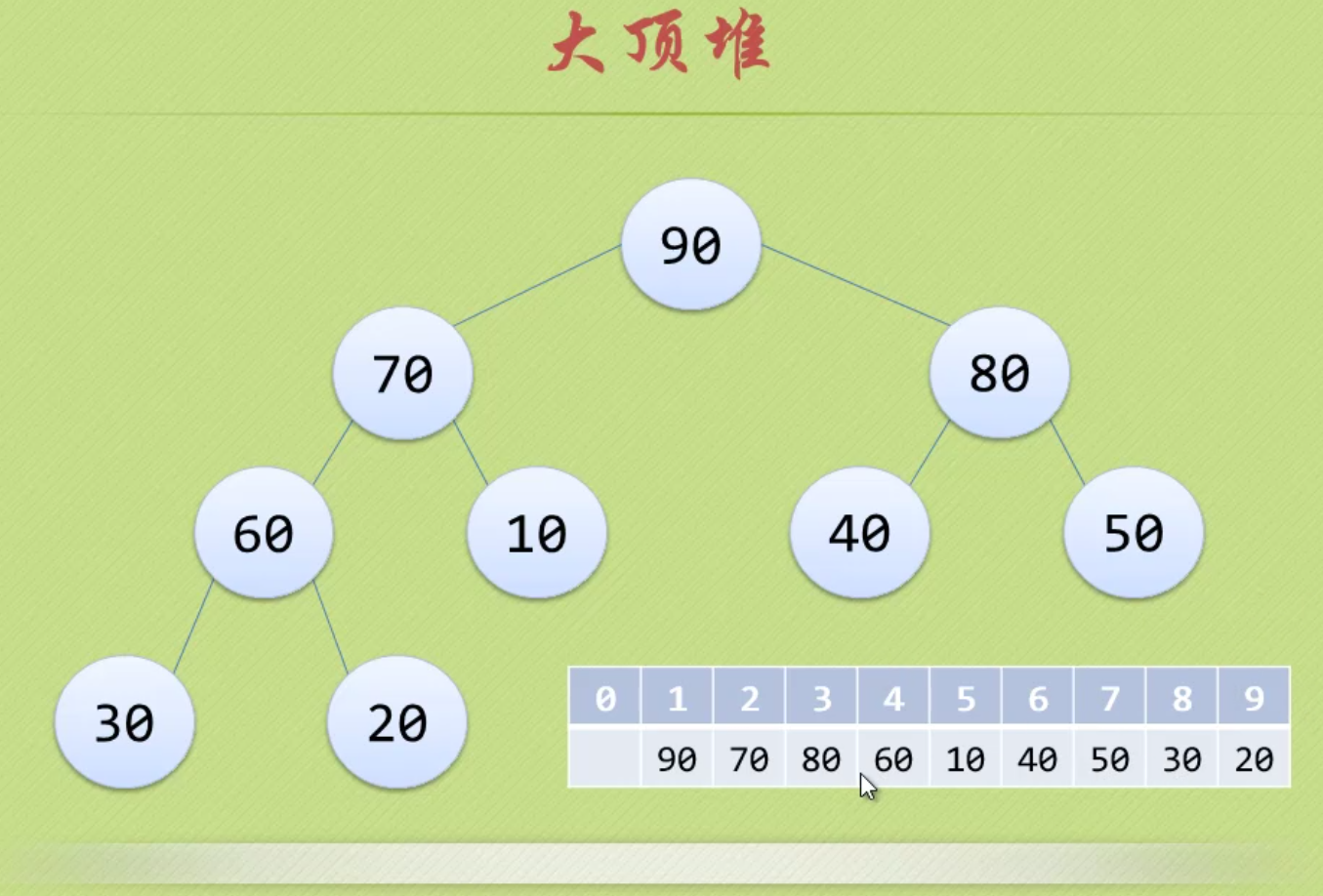注意！！！数组从1开始，1~n
void heapSort(int array[], int n)
{
int i;
for (i=n/2;i>0;i--)
{
}
for( i=n;i>1;i--)
{
swap(array, 1, i);
}
}
void HeapAdjust(int array[], int s, int n )
{
int i,temp;
temp = array[s];
for(i=2*s;i<=n;i*=2)
{
if(i<n&&array[i]<array[i+1])
{
i++;
}
if(temp>=array[i])
{
break;
}
array[s]=array[i];
s=i;
}
array[s]=temp;
}
void swap(int array[], int i, int j)
{
int temp;

temp=array[i];
array[i]=array[j];
array[j]=temp;
}
7 希尔排序
7.1 过程
希尔排序是插入排序改良的算法，希尔排序步长从大到小调整，第一次循环后面元素逐个和前面元素按间隔步长进行比较并交换，直至步长为1，步长选择是关键。
7.2 动图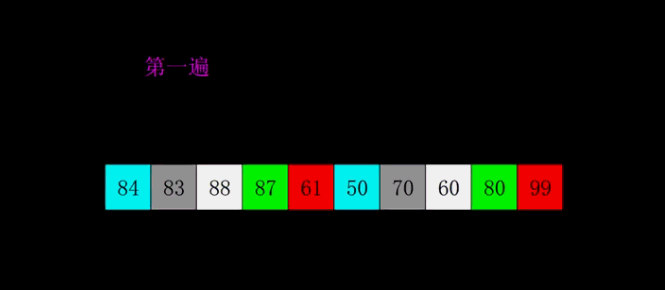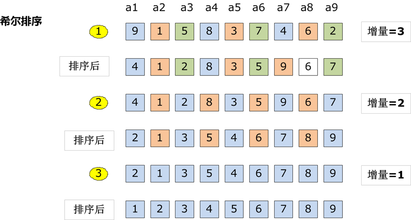7.3 核心程序（函数）
//下面是插入排序
void InsertSort( int array[], int n)
{
int i,j,temp;
for( i=0;i<n;i++ )
{
if(array[i]<array[i-1])
{
temp=array[i];
for( j=i-1;array[j]>temp;j--)
{
array[j+1]=array[j];
}
array[j+1]=temp;
}
}
}
//在插入排序基础上修改得到希尔排序
void SheelSort( int array[], int n)
{
int i,j,temp;
int gap=n; //~~~~~~~~~~~~~~~~~~~~~
do{
gap=gap/3+1;  //~~~~~~~~~~~~~~~~~~
for( i=gap;i<n;i++ )
{
if(array[i]<array[i-gap])
{
temp=array[i];
for( j=i-gap;array[j]>temp;j-=gap)
{
array[j+gap]=array[j];
}
array[j+gap]=temp;
}
}
}while(gap>1);  //~~~~~~~~~~~~~~~~~~~~~~

}
8 桶排序（基数排序和基数排序的思想）
8.1 过程
桶排序是计数排序的变种，把计数排序中相邻的m个”小桶”放到一个”大桶”中，在分完桶后，对每个桶进行排序（一般用快排），然后合并成最后的结果。
8.2 图解8.3 核心程序
#include <stdio.h>
int main()
{
int a,i,j,t;
for(i=0;i<=10;i++)
a[i]=0;  //初始化为0

for(i=1;i<=5;i++)  //循环读入5个数
{
scanf("%d",&t);  //把每一个数读到变量t中
a[t]++;  //进行计数(核心行)
}

for(i=0;i<=10;i++)  //依次判断a~a
for(j=1;j<=a[i];j++)  //出现了几次就打印几次
printf("%d ",i);

getchar();getchar();
//这里的getchar();用来暂停程序，以便查看程序输出的内容
//也可以用system("pause");等来代替
return 0;
}
9 计数排序
9.1 过程
算法的步骤如下：  - 找出待排序的数组中最大和最小的元素  - 统计数组中每个值为i的元素出现的次数，存入数组C的第i项  - 对所有的计数累加（从C中的第一个元素开始，每一项和前一项相加）  - 反向填充目标数组：将每个元素i放在新数组的第C(i)项，每放一个元素就将C(i)减去1
9.2 图解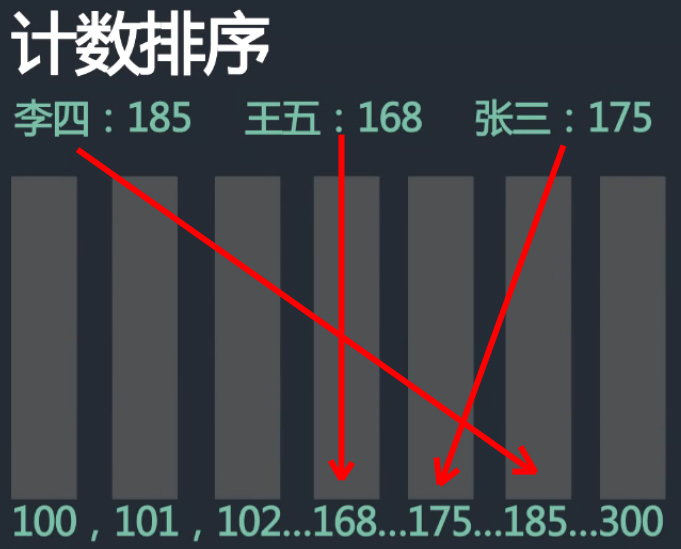9.3 核心程序（函数）
程序1：
#define NUM_RANGE (100)    //预定义数据范围上限，即K的值

void counting_sort(int *ini_arr, int *sorted_arr, int n)  //所需空间为 2*n+k
{
int *count_arr = (int *)malloc(sizeof(int) * NUM_RANGE);
int i, j, k;

//初始化统计数组元素为值为零
for(k=0; k<NUM_RANGE; k++){
count_arr[k] = 0;
}
//统计数组中，每个元素出现的次数
for(i=0; i<n; i++){
count_arr[ini_arr[i]]++;
}

//统计数组计数，每项存前N项和，这实质为排序过程
for(k=1; k<NUM_RANGE; k++){
count_arr[k] += count_arr[k-1];
}

//将计数排序结果转化为数组元素的真实排序结果
for(j=n-1 ; j>=0; j--){
int elem = ini_arr[j];          //取待排序元素
int index = count_arr[elem]-1;  //待排序元素在有序数组中的序号
sorted_arr[index] = elem;       //将待排序元素存入结果数组中
count_arr[elem]--;              //修正排序结果，其实是针对算得元素的修正
}
free(count_arr);
}

程序2：C++(最大最小压缩桶数)
public static void countSort(int[] arr) {
if (arr == null || arr.length < 2) {
return;
}
int min = arr;
int max = arr;
for (int i = 1; i < arr.length; i++) {
min = Math.min(arr[i], min);
max = Math.max(arr[i], max);
}
int[] countArr = new int[max - min + 1];
for (int i = 0; i < arr.length; i++) {
countArr[arr[i] - min]++;
}
int index = 0;
for (int i = 0; i < countArr.length; i++) {
while (countArr[i]-- > 0) {
arr[index++] = i + min;
}
}
10 基数排序
10.1 过程
基数排序是基于数据位数的一种排序算法。  它有两种算法  ①LSD–Least Significant Digit first 从低位（个位）向高位排。  ②MSD– Most Significant Digit first 从高位向低位（个位）排。  时间复杂度O(N*最大位数)。  空间复杂度O(N)。
10.2 图解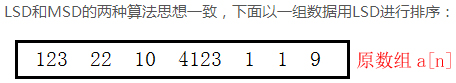对a[n]按照个位0~9进行桶排序：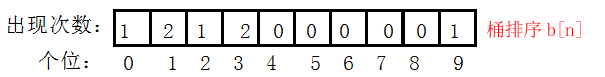对b[n]进行累加得到c[n]，用于b[n]中重复元素计数  ！！！b[n]中的元素为temp中的位置！！！跳跃的用++补上：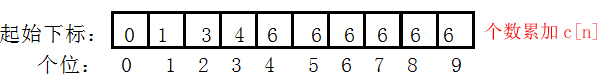temp数组为排序后的数组，写回a[n]。temp为按顺序倒出桶中的数据（联合b[n],c[n],a[n]得到），重复元素按顺序输出：10.3 核心程序
//基数排序
//LSD  先以低位排，再以高位排
//MSD  先以高位排，再以低位排
void LSDSort(int *a, int n)
{
assert(a);  //判断a是否为空，也可以a为空||n<2返回
int digit = 0;   //最大位数初始化
for (int i = 0; i < n; ++i)
{   //求最大位数
while (a[i] > (pow(10,digit)))  //pow函数要包含头文件math.h，pow(10,digit)=10^digit
{
digit++;
}
}
int flag = 1;   //位数
for (int j = 1; j <= digit; ++j)
{
//建立数组统计每个位出现数据次数（Digit[n]为桶排序b[n]）
int Digit = { 0 };
for (int i = 0; i < n; ++i)
{
Digit[(a[i] / flag)%10]++;  //flag=1时为按个位桶排序
}
//建立数组统计起始下标（BeginIndex[n]为个数累加c[n]，用于记录重复元素位置
//flag=1时，下标代表个位数值，数值代表位置，跳跃代表重复）
int BeginIndex = { 0 };
for (int i = 1; i < 10; ++i)
{
//累加个数
BeginIndex[i] = BeginIndex[i - 1] + Digit[i - 1];
}
//建立辅助空间进行排序
//下面两条可以用calloc函数实现
int *tmp = new int[n];
memset(tmp, 0, sizeof(int)*n);//初始化
//联合各数组求排序后的位置存在temp中
for (int i = 0; i < n; ++i)
{
int index = (a[i] / flag)%10;  //桶排序和位置数组中的下标
//计算temp相应位置对应a[i]中的元素，++为BeginIndex数组数值加1
//跳跃间隔用++来补，先用再++
tmp[BeginIndex[index]++] = a[i];
}
//将数据重新写回原空间
for (int i = 0; i < n; ++i)
{
a[i] = tmp[i];
}
flag = flag * 10;
delete[] tmp;
}
}  
附：
1 完整程序框架（冒泡排序举例）
1.1 VS2010程序
#include "stdafx.h"
#include "stdio.h"
#include <stdlib.h>

void BubbleSort(int array[], int n){
int i,j,k,count1=0, count2=0;
for(i=0; i<n-1; i++)
for(j=n-1; j>i; j--)
{
count1++;
if(array[j-1]>array[j])
{
count2++;
k=array[j-1];
array[j-1]=array[j];
array[j]=k;
}
}
printf("总共的循环次序为：%d,  总共的交换次序为：%d\n\n", count1, count2);
}

int main(int argc, _TCHAR* argv[])
{
int as[]={0,1,2,3,4,6,8,5,9,7};
BubbleSort(as, 10);
for(int i=0; i<10; i++)
{
printf("%d", as[i]);
}
printf("\n\n");
system("pause");
return 0;
}
1.2 执行程序（OJ）
#include <stdio.h>

void BubbleSort(int array[], int n){
int i,j,k,count1=0, count2=0;
for(i=0; i<n-1; i++)
for(j=n-1; j>i; j--)
{
count1++;
if(array[j-1]>array[j])
{
count2++;
k=array[j-1];
array[j-1]=array[j];
array[j]=k;
}
}
printf("总共的循环次序为：%d,  总共的交换次序为：%d\n\n", count1, count2);
}

int main()
{
int as[]={0,1,2,3,4,6,8,5,9,7};
BubbleSort(as, 10);
int i=0;
for(i=0; i<10; i++)
{
printf("%d", as[i]);
}
return 0;
}
2 关于交换的优化
不用中间变量进行交换
if(A[j] <= A[i]){
A[j] = A[j] + A[i];
A[i] = A[j] - A[i];
A[j] = A[j] - A[i];
}
3 C语言实现数组动态输入
#include <stdio.h>
#include <assert.h>  //断言头文件
#include <stdlib.h>

int main(int argc, char const *argv[])
{
int size = 0;
scanf("%d", &size);   //首先输入数组个数
assert(size > 0);     //判断数组个数是否非法

int *array = (int *)calloc(size, sizeof(int));  //动态分配数组
if(!R1)
{
return;           //申请空间失败
}

int i = 0;
for (i = 0; i < size; ++i) {
scanf("%d", &array[i]);
}

mergeSort(array, size);
printArray(array, size);

free(array);
return 0;
} 
注：  1.colloc与malloc类似,但是主要的区别是存储在已分配的内存空间中的值默认为0,使用malloc时,已分配的内存中可以是任意的值.  2.colloc需要两个参数,第一个是需要分配内存的变量的个数,第二个是每个变量的大小.
展开全文• 关于算法时间复杂度的计算 关于算法时间复杂度的计算 关于算法时间复杂度的计算
• 数据结构和算法-1-算法的引入和算法时间复杂度.pdf
• 1 常用算法时间复杂度 来源： http://www.bigocheatsheet.com/ 1.1 常用数据结构算法复杂度 1.2 常用排序算法复杂度
1 常用算法时间复杂度
来源： http://www.bigocheatsheet.com/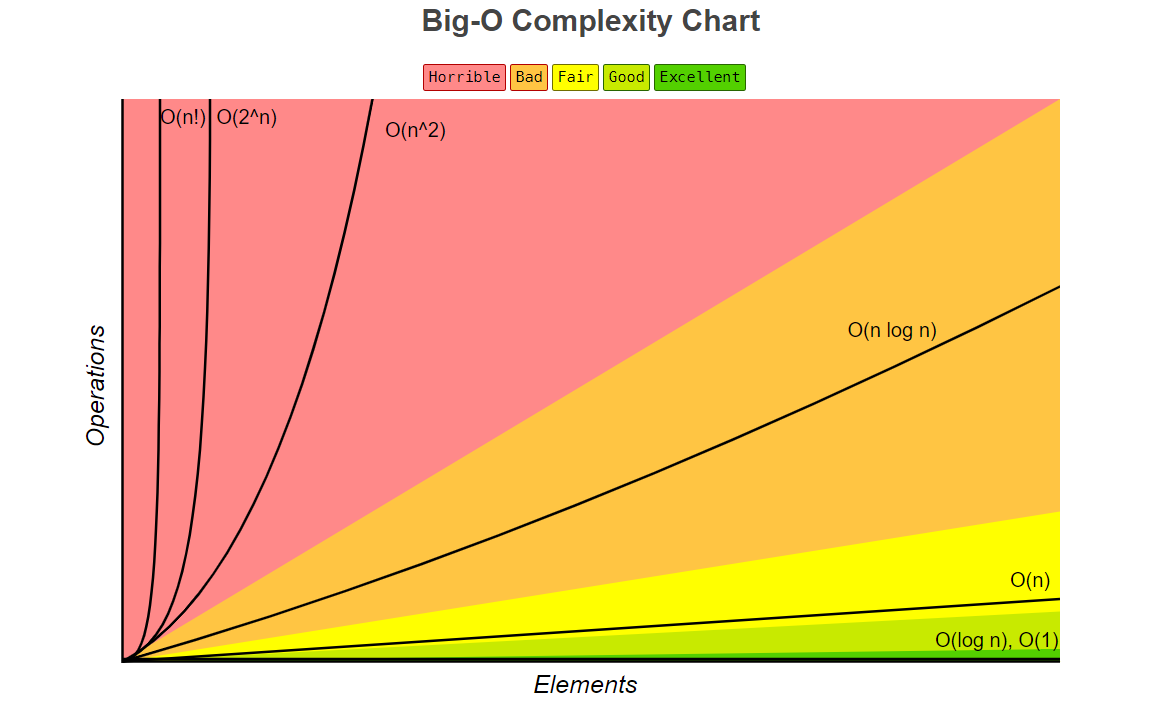1.1 常用数据结构算法复杂度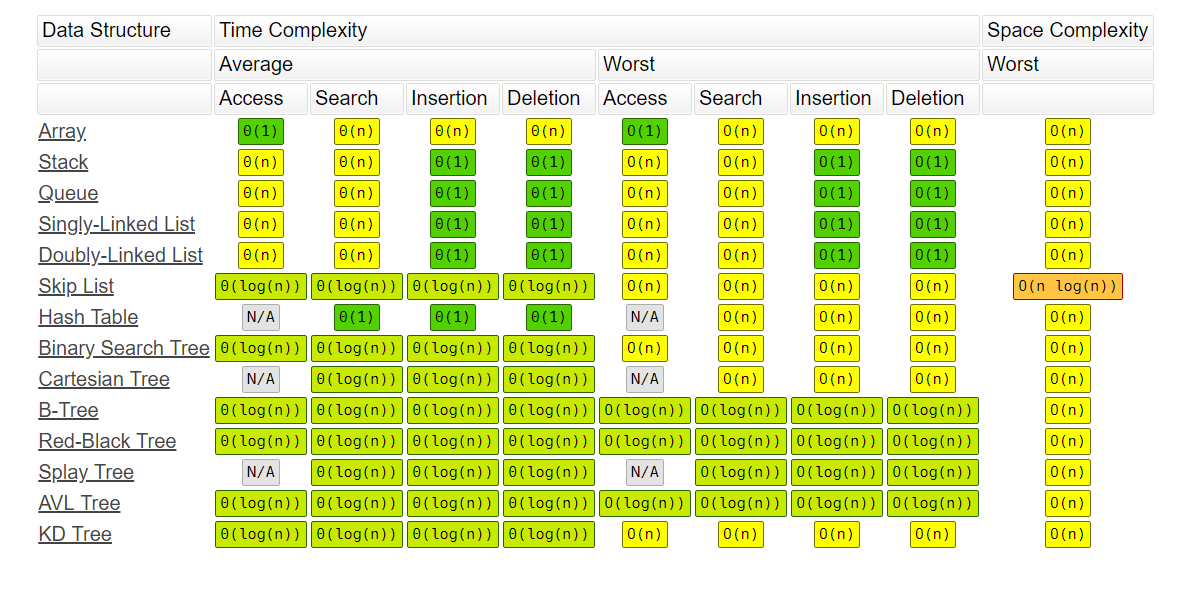1.2 常用排序算法复杂度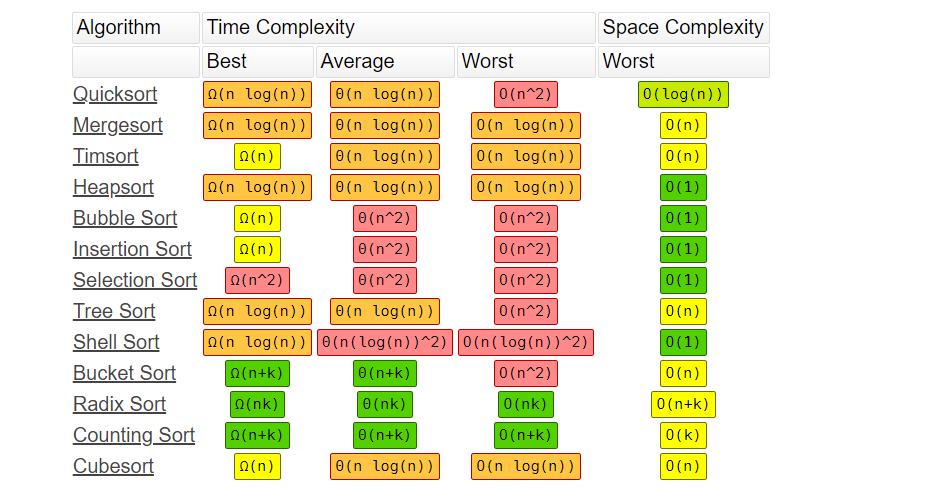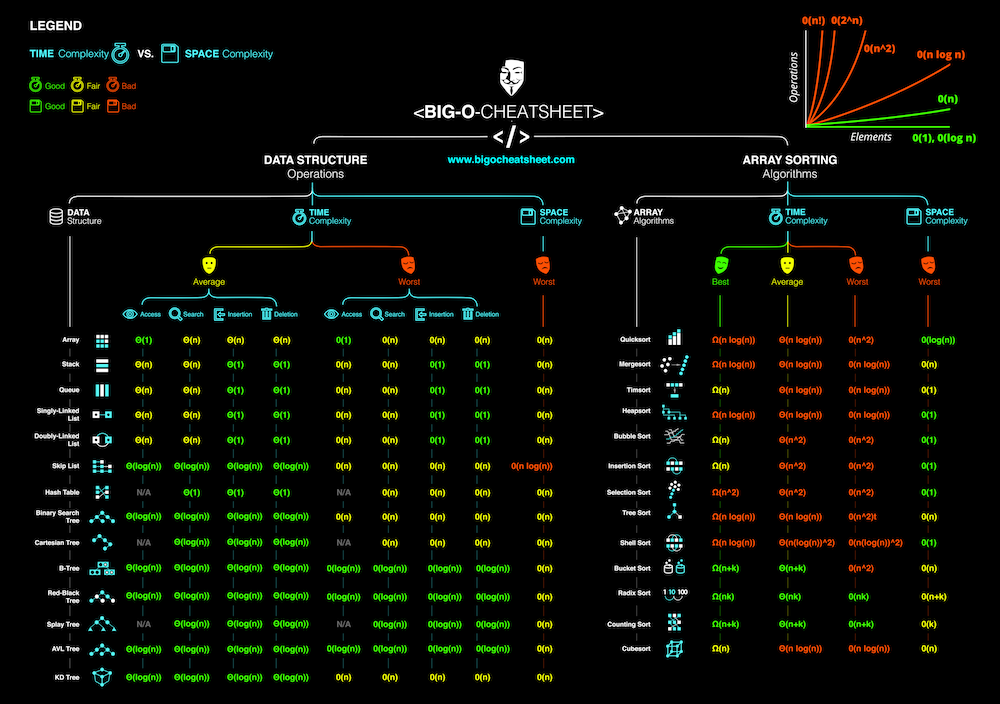展开全文• 算法时间复杂度分析 将算法中基本操作的执行次数作为算法时间复杂度的度量； （换句话说： 算法中基本操作的重复执行的次数就是算法的计算量，将其大小作为算法的时间复杂度 注：语句的频度指的是该语句重复执行...c
• 大学二年级课程 算法设计与分析的一般算法时间复杂度的证明过程,希望可以帮到大家.
• 算法时间复杂度：讲算法中基本操作的次数作为算法时间复杂度的度量 void fun(int n) { int i=0;s=0; while(s) { ++i; s=s+i; } }求算法时间复杂度首先找出基本操作，有函数看出，++i,s=s+i属于基本操作，然后...
• 算法-数据结构和算法-1-算法的引入和算法时间复杂度.rar
•数据结构
•排序算法空间复杂度 排序算法稳定性
• 算法时间复杂度分析基础算法时间复杂度分析基础算法时间复杂度分析基础
• 之前一直默认Dijkstra算法时间复杂度为 o(n2)o(n^{2})o(n2)，没有思考过具体的时间复杂度，今天把这个弄清楚。 Dijkstra算法的思路与关键点 思路：广度优先 + 松弛 所有点分为两个集合SSS和TTT，SSS最开始只包括...堆栈
• 定义：在进行算法分析时，语句总的执行次数T(n)是关于问题规模n的函数，进行分析T(n)随着n的变化情况并确定T(n)的数量级。算法的时间复杂度，也就是算法的时间度量，记作：T...这样用大写O()来体现算法时间复杂度的记法...